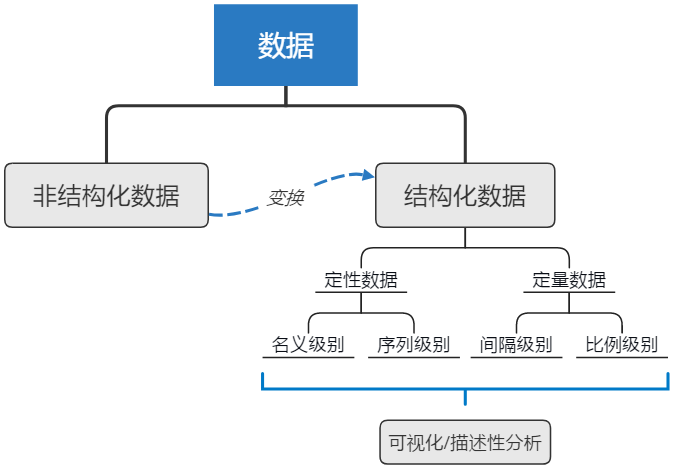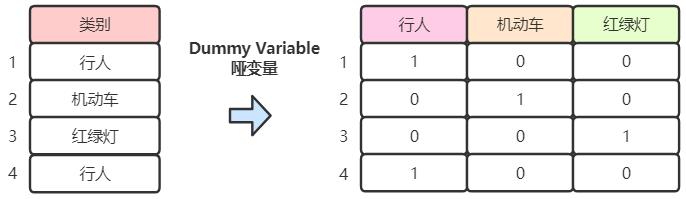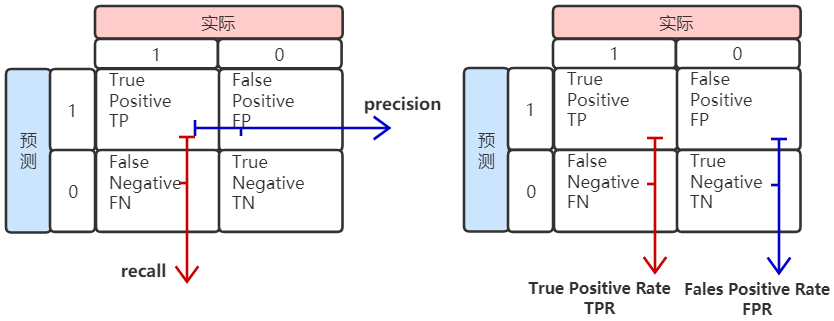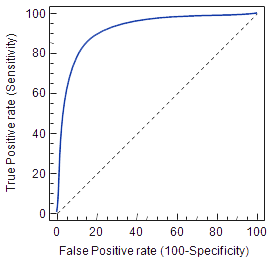# 机器学习中的特征工程详解

### 1 特征认识（Feature understanding）

• 结构化数据：可分解为观测记录和属性的数据，如表格数据，行为观测，列为属性
• 非结构化数据：数据形式随意，不遵循特定规则，如一堆数据（log文件），博客信息，或者只有一个特征的数据
• 定量数据：有明确数值的数据
• 定性数据：类别数据

##### 1.2 四种数据级别
• 名义级别（The nominal level）：全部为定性数据，不能进行加减乘除数学运算，但可以做计数（count）统计，如血型数据，可采用饼图、直方图可视化
• 序列级别（The ordinal level）：与名义级别基本一致，但数据可自然排序，为类别（category）数据，数学上数据可比较（compare），如大学成绩A、B、C、D，可采用Box-plot可视化
• 间隔级别（The interval level）：除了名义级别和序列级别的特性，间隔级别数据还可进行加减运算，如温度
• 比例级别（The ratio level）：除了上述级别的特性，比例级别可进行乘除运算（拥有0物理含义），如重量，钱，100块是50块的2倍### 2 特征改进（Feature improvement）

##### 2.1 缺失值处理
• 删除缺失值记录：调用pandas包的dropna()
• （定量数据）填充均值或中值：（pandas包）data.fillna(value)，（sklearn包）Imputer().fit_transform(data)
• （定性数据）填充众数：（pandas包）data.fillna(data.value_counts().index)

##### 2.2 标准化和正态化（Standardization and Normalization）
• Z-score standardization：$$z=(x-\mu )/\sigma$$，sklearn包调用StandardScaler().fit_transform(data)
• Min-max scaling：$$m=(x-min)/(max-min)$$，sklearn包调用MinMaxScaler().fit_transform(data)
• Row normalization：使每一行数据的L2范数（L2 Norm）$$\left| x \right|=\sqrt{(x_{1}^{2}+x_{2}^{2}+\cdots +x_{n}^{2})}$$一致，sklearn包调用Normalizer().fit_transform(data)

### 3 特征构建（Feature Construction）

from sklearn.base import TransformerMixin

class CustomCategoryImputer(TransformerMixin):
def __init__(self, cols=None):
self.cols = cols

def transform(self, df):
X = df.copy()
for col in self.cols:
X[col].fillna(X[col].value_counts().index, inplace=True)
return X

def fit(self, *_):
return self

cci = CustomCategoryImputer(cols=['col1', 'col2'])
imputer = Pipeline([('category', cci), ('xxx', xxx)])
imputer.fit_transform(X)
##### 3.1 类别数据编码（Encoding categorical variables）
• 名义级别编码：将类别数据转换为哑变量（Dummy Variable），如（性别：男or女）=>（男：0 or 1；女：0 or 1）（注意这个例子中其实（女：0 or 1）为多余的），类似于One-hot编码，在pandas中可调用get_dummies()
• 序列级别编码：可采用标签编码（Label Encoder），将序列按0~(n-1)编码
• 数值特征组合：有时也可将数值特征按多项式组合，生成新的特征，可调用sklearn包的PolynomialFeatures()##### 3.2 文字特征编码

• 标记（Tokenizing）：将一段文字（英文）按标点符号和空格进行分割，获取标记单词
• 计数（Counting）：统计标记出现的频率
• 标准化（Normalizing）：消除文字长度对标记重要性的影响

### 4 特征选择

##### 4.1 模型性能评估指标

• 精确率（precision）：TP / (TP+FP)
• 召回率（recall）：TP / (TP+FN)
• 准确率（accuracy，ACC）：(TP + TN) / (TP + TN + FP + FN)
• F1 Score：精准率和召回率的调和均值，2 / F1 = 1 / P + 1 / R• True Positive Rate: TPR = TP / (TP+FN) → 将正例分对的概率
• Fales Positive Rate: FPR = FP / (FP+TN) → 将负例错分为正例的概率
• ROC曲线：在ROC空间中，每个点的横坐标是FPR，纵坐标是TPR，ROC曲线越靠近左上角，模型越好。ROC曲线有个很好的特性：当测试集中的正负样本的分布变化的时候，ROC曲线能够保持不变，解决类不平衡（class imbalance）的问题
• AUC：ROC曲线下的面积，AUC越接近1模型越好• $$R^2$$
• 平均绝对误差MAE（Mean Absolute Error）：L1范数损失
• 平均平方误差MSE（Mean Squared Error）：L2范数损失

• 数据训练时间
• 数据预测时间
• 所需的计算机内存等
##### 4.2 特征选择方法

（1）基于概率统计理论的特征选择

• 根据特征与预测目标的相关性系数挑选
from sklearn.base import TransformerMixin, BaseEstimator
class CustomCorrelationChooser(TransformerMixin, BaseEstimator):
def __init__(self, response, cols_to_keep=[], threshold=None):
self.response = response
self.threshold = threshold
self.cols_to_keep = cols_to_keep

def transform(self, X):
return X[self.cols_to_keep]

def fit(self, X, *_):
df = pd.concat([X, self.response], axis=1)
self.cols_to_keep = df.columns[df.corr()[df.columns[-1]].abs() > self.threshold]
self.cols_to_keep = [c for c in self.cols_to_keep if c in X.columns]
return self
• 采用不同假设检验方法挑选特征，如ANOVA、Chi2检验等
from sklearn.feature_selection import SelectKBest
from sklearn.feature_selection import f_classif
k_best = SelectKBest(f_classif, k=5)
k_best.fit_transform(X, y)
p_values = pd.DataFrame({'column': X.columns, 'p_value': k_best.pvalues_}).sort_values('p_value')
p_values[p_values['p_value'] < .05]

（2）基于机器学习模型的特征选择

• 根据决策树一类模型的特征选择
from sklearn.feature_selection import SelectFromModel
select_from_model = SelectFromModel(DecisionTreeClassifier(), threshold=.05)
selected_X = select_from_model.fit_transform(X, y)
• 根据线性模型的特征选择，如线性回归、逻辑回归、支持向量机等，引入正则项能防止过拟合，提高模型泛化能力
• L1正则（lasso正则）
• L2正则（ridge正则）
from sklearn.feature_selection import SelectFromModel
logistic_selector = SelectFromModel(LogisticRegression(penalty=l1), threshold=.05)
selected_X = logistic_selector.fit_transform(X, y)
##### 4.3 特征选择经验
• 如果大部分特征为类别特征，可采用Chi2检验或者决策树之类的模型进行选择
• 如果大部分特征为数值特征，可采用线性模型进行选择
• 如果为二分类问题，采用SelectFromModel()函数和支持向量机模型
• 有些情况下，也可进行探索性数据分析，手工选择特征，或根据专业知识选择特征

### 5 特征变换（Feature Transformation）

• 主成分分析（principal components analysis）：sklearn包的PCA
• 线性判别分析（linear discriminant analysis）：sklearn包的LinearDiscriminantAnalysis

PCA是从特征的协方差角度，希望主成分方向携带尽量多的信息量；而LDA则是在已知样本的类标注， 希望投影到新的基后使得不同的类别之间的数据点的距离更大，同一类别的数据点更紧凑。

### 6 特征学习（Feature Learning）

• 受限玻尔兹曼机（Restricted Boltzmann Machine，RBM）：包含两层的神经网络，可见层（visible layer）和隐藏层（hidden layer），可见层神经元数量等于输入的特征数，隐藏层神经元数量等于希望提取的特征数量，sklearn包的BernoulliRBM
##### 6.1 文字特征学习
• Word2Vec
• GloVe

05-22160104-1570
11-133668
06-124505
08-201万+
10-252万+
04-081万+
06-27418
10-162413